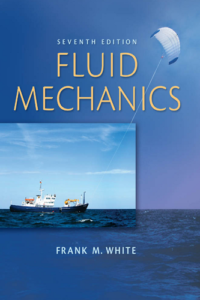اسم المؤلف
Frank M. White
التاريخ
9 أكتوبر 2016
المشاهدات
314
التقييمLoading...

Fluid Mechanics 7th – Seventh Edition
Frank M. White
University of Rhode Island
Preface xi
Chapter 1
Introduction 3
1.1 Preliminary Remarks 3
1.2 History and Scope of Fluid Mechanics 4
1.3 Problem-Solving Techniques 6
1.4 The Concept of a Fluid 6
1.5 The Fluid as a Continuum 8
1.6 Dimensions and Units 9
1.7 Properties of the Velocity Field 17
1.8 Thermodynamic Properties of a Fluid 18
1.9 Viscosity and Other Secondary Properties 25
1.10 Basic Flow Analysis Techniques 40
1.11 Flow Patterns: Streamlines, Streaklines, and
Pathlines 41
1.12 The Engineering Equation Solver 46
1.13 Uncertainty in Experimental Data 46
1.14 The Fundamentals of Engineering (FE)
Examination 48
Problems 49
Fundamentals of Engineering Exam Problems 57
Comprehensive Problems 58
References 61
Chapter 2
Pressure Distribution in a Fluid 65
2.1 Pressure and Pressure Gradient 65
2.2 Equilibrium of a Fluid Element 67
2.3 Hydrostatic Pressure Distributions 68
2.4 Application to Manometry 75
2.5 Hydrostatic Forces on Plane Surfaces 78
2.6 Hydrostatic Forces on Curved Surfaces 86
2.7 Hydrostatic Forces in Layered Fluids 89
2.8 Buoyancy and Stability 91
2.9 Pressure Distribution in Rigid-Body Motion 97
2.10 Pressure Measurement 105
Summary 109
Problems 109
Word Problems 132
Fundamentals of Engineering Exam
Problems 133
Comprehensive Problems 134
Design Projects 135
References 136
Chapter 3
Integral Relations for a Control Volume 139
3.1 Basic Physical Laws of Fluid Mechanics 139
3.2 The Reynolds Transport Theorem 143
3.3 Conservation of Mass 150
3.4 The Linear Momentum Equation 155
3.5 Frictionless Flow: The Bernoulli Equation 169
3.6 The Angular Momentum Theorem 178
3.7 The Energy Equation 184
Summary 195
Problems 195
Word Problems 224
Fundamentals of Engineering Exam Problems 224
Comprehensive Problems 226
Design Project 227
References 227
Contents
Chapter 4
Differential Relations for Fluid Flow 229
4.1 The Acceleration Field of a Fluid 230
4.2 The Differential Equation of Mass Conservation 232
4.3 The Differential Equation of Linear Momentum 238
4.4 The Differential Equation of Angular Momentum 244
4.5 The Differential Equation of Energy 246
4.6 Boundary Conditions for the Basic Equations 249
4.7 The Stream Function 253
4.8 Vorticity and Irrotationality 261
4.9 Frictionless Irrotational Flows 263
4.10 Some Illustrative Incompressible Viscous Flows 268
Summary 276
Problems 277
Word Problems 288
Fundamentals of Engineering Exam Problems 288
Comprehensive Problems 289
References 290
Chapter 5
Dimensional Analysis and Similarity 293
5.1 Introduction 298
5.2 The Principle of Dimensional Homogeneity 296
5.3 The Pi Theorem 302
5.4 Nondimensionalization of the Basic Equations 312
5.5 Modeling and Its Pitfalls 321
Summary 333
Problems 333
Word Problems 342
Fundamentals of Engineering Exam Problems 342
Comprehensive Problems 343
Design Projects 344
References 344
Chapter 6
Viscous Flow in Ducts 347
6.1 Reynolds Number Regimes 347
6.2 Internal versus External Viscous Flow 352
6.3 Head Loss—The Friction Factor 355
6.4 Laminar Fully Developed Pipe Flow 357
6.5 Turbulence Modeling 359
6.6 Turbulent Pipe Flow 365
6.7 Four Types of Pipe Flow Problems 373
6.8 Flow in Noncircular Ducts 379
6.9 Minor or Local Losses in Pipe Systems 388
6.10 Multiple-Pipe Systems 397
6.11 Experimental Duct Flows: Diffuser Performance 403
6.12 Fluid Meters 408
Summary 429
Problems 430
Word Problems 448
Fundamentals of Engineering Exam Problems 449
Comprehensive Problems 450
Design Projects 452
References 453
Chapter 7
Flow Past Immersed Bodies 457
7.1 Reynolds Number and Geometry Effects 457
7.2 Momentum Integral Estimates 461
7.3 The Boundary Layer Equations 464
7.4 The Flat-Plate Boundary Layer 467
7.5 Boundary Layers with Pressure Gradient 476
7.6 Experimental External Flows 482
Summary 509
Problems 510
Word Problems 523
Fundamentals of Engineering Exam Problems 524
Comprehensive Problems 524
Design Project 525
References 526
Chapter 8
Potential Flow and Computational Fluid Dynamics 529
8.1 Introduction and Review 529
8.2 Elementary Plane Flow Solutions 532
8.3 Superposition of Plane Flow Solutions 539
8.4 Plane Flow Past Closed-Body Shapes 545
8.5 Other Plane Potential Flows 555
8.6 Images 559
8.7 Airfoil Theory 562
8.8 Axisymmetric Potential Flow 574
8.9 Numerical Analysis 579
Summary 593
Problems 594
Word Problems 604
Comprehensive Problems 605
Design Projects 606
References 606
Chapter 9
Compressible Flow 609
9.1 Introduction: Review of Thermodynamics 609
9.2 The Speed of Sound 614
9.4 Isentropic Flow with Area Changes 622
9.5 The Normal Shock Wave 629
9.6 Operation of Converging and Diverging Nozzles 637
9.7 Compressible Duct Flow with Friction 642
9.8 Frictionless Duct Flow with Heat Transfer 654
9.9 Two-Dimensional Supersonic Flow 659
9.10 Prandtl-Meyer Expansion Waves 669
Summary 681
Problems 682
Word Problems 695
Fundamentals of Engineering Exam Problems 696
Comprehensive Problems 696
Design Projects 698
References 698
Chapter 10
Open-Channel Flow 701
10.1 Introduction 701
10.2 Uniform Flow: The Chézy Formula 707
10.3 Efficient Uniform-Flow Channels 712
10.4 Specific Energy: Critical Depth 714
10.5 The Hydraulic Jump 722
10.7 Flow Measurement and Control by Weirs 734
Summary 741
Problems 741
Word Problems 754
Fundamentals of Engineering Exam Problems 754
Comprehensive Problems 754
Design Projects 756
References 756
Chapter 11
Turbomachinery 759
11.1 Introduction and Classification 759
11.2 The Centrifugal Pump 762
11.3 Pump Performance Curves and Similarity Rules 76
11.4 Mixed- and Axial-Flow Pumps: The Specific Speed
11.5 Matching Pumps to System Characteristics 785
11.6 Turbines 793
Summary 807
Problems 807
Word Problems 820
Comprehensive Problems 820
Design Project 822
References 822
Appendix A Physical Properties of Fluids 824
Appendix B Compressible Flow Tables 829
Appendix C Conversion Factors 836
Appendix D Equations of Motion in Cylindrical Coordinates